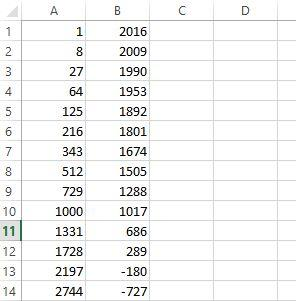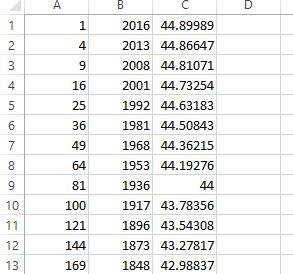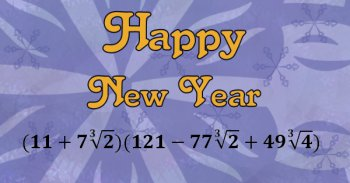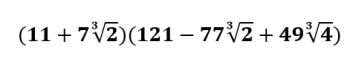Games
Problems
Go Pro!

Category results for 'new-year-number'.

Lavinia from Italy writes: "Hi professor. I have just read your post about this year's number (2017). In this post you say that we can write 2017 as a the sum of one cube plus twice another. 2017 = 11+ 2 · 73 I was wondering how you managed manage to discover this. Did you use a formula or something? Thanks, Lavinia"

Thanks for asking, Lavinia. For those who missed it, this is a reference to the following blog post: Happy New Year 2017. In this blog post I make mention of the fact Lavinia pointed out:

2017 = 11+ 2 · 73

So how did I discover this odd fact? There wasn't a formula. I found it simply by playing around with the numbers to see what interesting things I found. I don't remember exactly what I was doing when I discovered that, but it probably involved a spreadsheet. I probably was thinking, "I wonder what results I'll get if I subtract perfect cubes from 2017!"

So I probably entered the following formula into cell A1 of a spreadsheet:

= ROW()^3 (cube the row number)

And then, in B1 cell put the following formula:

= 2017 - A1

Then I would have used the "fill down" feature to populate a bunch of rows with the results of those calculations:As I looked down through this, I'm sure one thing that caught my eye was the fact that the 11th row contained two palindromes (which I mentioned in the blog post). Once I'd made that observation, I would have been curious to see how 686 factored, which would have led to the equation you asked about.

Incidentally, this time as I looked at the rows of numbers, I realized that there's a perfect square in the second column: 289 = 172. So we can also write:

2017 = 12+ 172

I probably did something very similar to find the perfect squares that add to 2017:

A1 = ROW()^2
B1 = 2017 - A1
C1 = SQRT(B1)

This would have given the following:From there, it's very easy to see that there's a number in column C that doesn't have a decimal, which is how I knew that I'd found a pair of perfect squares that add to 2017:

2017 = 92 + 442

Incidentally, there was another row that had no decimal, further down the spreadsheet. It was row number 44, of course!

Excel (or any other spreadsheet application) can be a great help in exploring mathematical oddities. I realize that I'm a bit spoiled, having calculators and spreadsheets to use when playing with numbers. Imagine trying to figure out quirky number facts like these without even a calculator!

It's my yearly tradition to take the number for the new year and see if I can find interesting mathematical novelties about the new year.The first thing I always do is check the prime factorization. It turns out 2017 is a prime number. So that's something novel, yet not extremely unusual, since there are an infinite number of primes. The previous prime year was 2011, and the next one is 2027.

Incidentally, since it is a prime, and it is one more than a multiple of four, Fermat's theorem on the Sums of Two Squares gives us an interesting conclusion: 2017 can be written as the sum of two perfect squares.

The two squares, in case you were wondering, are the squares of 9 and 44. 2017 = 92 + 442.

Can it be written as the sum of three perfect squares? Yes it can, as follows:

122 + 282 + 332
182 + 182 + 372
212 + 262 + 302

For those who enjoy numerology, multiply the two numbers being squared in the second row above (18 and 37), and you'll get a beastly number (666). And you thought 2016 was bad.

Not that this is terribly unusual, but 2017 can be written as the sum of two palindromes:

2017 = 1331 + 686

Of course, I also like to see if I can find a ridiculously ugly way of writing it, and this year's looks like this:That looks appalling, I know. You're welcome to multiply it out, and you'll see that it works out to 2017. If you're wondering how I came up with that nasty thing, I did it by noticing the following:

2017 = 1331 + 686 (as mentioned above)
2017 = 113 + 2×73
2017 = 113 + (7×21/3)3

And then it was just a matter of using the sum of cubes factoring rule:

a3 + b3 = (a + b)(a2 - ab + b2)

It's interesting that 2017 can be written as a perfect square plus twice another perfect square (2017 = 372 + 2×182) and it can be written as a perfect cube plus twice another perfect cube (2017 = 113 + 2×73).

Happy New Year!

PS - if you write your dates as m/d/y, you'll be looking forward to July 10, 2017, which is 7102017, another palindrome. Otherwise you'll be looking forward to October 7, 2017.One of the first things I do every year when I realize that the new year upon us...

...no, not New Year's resolutions.

No, I always reduce the new year to its prime factorization. Why? Because sometimes I write competition math problems involving the school year, and so it's nice to know how the number factors.

In case you were wondering, 2016 = 25327.

It's also, by the way, 25 less than 211. That's right - in 32 more years, the year number will be a perfect 11th power.

And if you want to verify that without using a calculator:

25327 + 25 = 25(327 + 1) = 25(63 + 1) = 2526 = 211.

Obviously, even when I'm on school vacation, my math mind doesn't shut down. In fact, I just spent a good part of a day writing a math unit that some of my high school students will be working on later on this year: Probability. This unit covers essential concepts like dependent and independent events, mutually exclusive outcomes, complementary outcomes, and compound events. There may eventually be a second probability unit that delves into more topics, such as conditional probability. For now, if you're looking for basic concepts, this is a good starting point.

Meanwhile, a unit on Pythagorean Means has also been added to the site.

It seems that some of you haven't left behind your math brains while on Christmas vacation, either. Professor Puzzler has fielded a handful of math-related questions while on vacation:

Thanks for all the great questions!

Happy 211 - 25,
Douglas TwitchellLike us on Facebook to get updates about new resources